[BACK]
 Intelligent Automation & Soft ComputingDOI:10.32604/iasc.2021.018289Article

Optimal Parameter Estimation of Proton Exchange Membrane Fuel Cells

1Mechanical Engineering Department, College of Engineering & Islamic Arch., Umm Al-Qura University, Makkah, Saudi Arabia
2College of Engineering at Wadi Addawaser, Prince Sattam Bin Abdulaziz University, 11911 Al-Kharj, Saudi Arabia
3Electrical Engineering Department, Faculty of Engineering, Minia University, 61517, Minia, Egypt
4Production Engineering and Design Department, Faculty of Engineering, Minia University, 61517, Minia, Egypt
5Mechanical Engineering Department, Faculty of Engineering, Sohag University, Sohag, Egypt
*Corresponding Author: Hegazy Rezk. Email: hr.hussien@psau.edu.sa
Received: 04 March 2021; Accepted: 09 April 2021

Abstract: The problem of parameter estimation of the proton exchange membrane fuel cell (PEMFC) model plays a significant role in the simulation and optimization of a PEMFC system. In the current research, a moth flame optimization algorithm (MFOA) is used to identify the best parameters of PEMFC. Two different PEMFCs, Nedstack PS6, 6 kW, and SR-12 PEM 500 W are used to demonstrate the accuracy of the MFOA. Throughout the optimization process, seven unidentified parameters (1, 2, 3, 4, λ, ℛ, and B) of PEMFC are appointed to be decision variables. The fitness function, which needed to be minimum, is represented by the root-mean-square error between the calculated voltage of the PEMFC and the experimental dataset. The results attained by the MFOA are compared with the sine cosine algorithm (SCA) and particle swarm optimization (PSO). The following metrics are considered in the comparison: best value, worst value, average value, standard deviation, and efficiency. The main findings verified the supremacy of the MFOA in estimating the best parameters of the PEMFC model in comparison with PSO and SCA. For the Nedstack PS6, the efficiency values are 24.50458%, 79.477%, and 38.84747% for MFOA, PSO and SCA, respectively. For PEMFC (SR-12 PEM), the average efficiency values are 14.965%, 79.933% and 31.87% for MFOA, PSO, and SCA, respectively.

Keywords: Parameter estimation; fuel cell; optimization; renewable energy

1  Introduction

The increasing global demand for low carbon-emitting fuel cell-based vehicles and growing adoption of clean sources of energy production have augmented the need for developments in fuel cell technology . Furthermore, increasing power sector and augmented distributed power generation capacities globally will further support the development of the fuel cell market . A fuel cell stack is an electrochemical device that converts the chemical energy of the fuel (most often hydrogen) into electrical energy . By application, the fuel cells are divided into transportation, portable, and stationary applications. Advances in fuel cell technology are occurring rapidly in the transportation sector due to the extensive use of fuel cells in heavy-duty land vehicles, such as public transportation buses. In addition, stationary fuel cell power systems are gaining more attention in commercial and residential buildings. Stationary fuel cells can be used in several applications such as supplying co-generation power, combined heat and power, off-grid primary source power systems, and backup power sources . Fuel cells are classified into proton exchange membrane fuel cells (PEMFC), phosphoric acid fuel cells (PAFC), alkaline fuel cells (AFC), and microbial fuel cell (MFC) . PEMFCs are responsible for most of the developments in fuel cell technology in 2020 and are expected to increase at the highest rate from 2021 to 2025 as a result of their applications in fuel cell-based electric vehicles.

An accurate model of a PEMFC depends on the availability of parameters that are unavailable in the manufacturing datasheet. It is therefore mandatory to determine the unknown PEMFC parameters to provide an accurate model closely matching the experimental data under various operational conditions .

The output voltage of a PEMFC relies on parameters related to the chemical procedures occurring inside the PEMFC . Some approaches are utilized to estimate the correct parameters of PEMFC. Among these approaches, modern optimization-based methods are employed to solve the PEMFC parameter identification problem due to their consistency, robustness, and simplicity. For example, Qin et al.  proposed an improved fluid search optimization algorithm to solve the problem of PEMFC. Additionally, Yuan et al.  suggested an optimized technique to estimate PEMFC parameters using an enhanced edition of the sunflower optimization algorithm. Cao et al.  introduced a linear–quadratic–Gaussian optimal process to control the PEMFC system. To enhance the performance of the control system, a modified version of the whale optimization algorithm has been used. El-Hay et al.  proposed a method based on an interior search algorithm to determine the steady-state and transient parameters of fuel cells. In the study, a proportional-integral controller is integrated with the dynamic model to enhance its performance throughout transient disturbances . Meanwhile, Menesy et al.  suggested an efficient parameter extraction strategy based on a modified artificial ecosystem optimizer applied to the model of PEMFC. The cost function is represented by the sum of squared errors between the measured and estimated voltage of the fuel cell.

In this work, a moth flame optimization algorithm (MFOA) is used to identify the best parameters of a PEMFC. Two different PEMFCs (NedStack PS6 and SR-12 PEM 500 W) are used to demonstrate the accuracy of the MFOA. Throughout the optimization process, seven unidentified parameters (1, 2, 3, 4, λ, ℛ, and B) of a PEMFC are appointed to be decision variables. The objective function, which needs to be the minimum, is represented by the root-mean-square error (RMSE) between the calculated voltage of a PEMFC and the experimental dataset. The results attained by the MFOA are compared with the sine cosine algorithm (SCA) and particle swarm optimization (PSO).

The contributions of the current work are briefly summed as follows:

•   MFOA is applied to estimate the best parameters of the PEMFC model.

•   A comprehensive comparison is made with PSO and SCA.

•   The superiority and reliability of the MFOA-based strategy in solving the PEMFC parameter identification problem is validated.

The rest of the paper is organized as follows. Section 2 presents a general description of the parameter identification problem of PEMFCs. A brief overview of the core idea of the MFOA, PSO, and the SCA are introduced in Section 3. Section 4 discusses the obtained results. Finally, the main findings are outlined in Section 5.

2  Problem Formulation

Details of the steady-state characteristics of PEMFC are described using electrochemical relations . Fig. 1a shows the structure of a PEMFC. The PEMFC model includes seven undetermined parameters. It is mandatory to correctly identify these parameters to obtain polarization curves that correspond to the experimental data. 1, 2, 3, and 4 denote the semi-empirical parametric factors of the PEMFC, with λ representing a scalar factor varying from 10 to 24, ℛ denoting the contact resistance (Ohm), and B representing a parametric coefficient (volt).Figure 1: (a) PEMFC structure, (b) output voltage of PEMFC against the current density

The output voltage of a PEMFC versus the current density is plotted in Fig. 1b. To estimate the output voltage ( Vout ), three voltage drops must be considered : activation, ohmic, and concentration voltage drops. The following relationship represents the output voltage of a PEMFC:

Vout=ENVaVoVc (1)

where Va , Vo , and Vc are the activation voltage drop, ohmic voltage drop, and concentration voltage drop, respectively.

The thermodynamic potential ( EN ) can be calculated using the following relationship:

EN=1.2290.85×103×(T298.15)+4.3085×105×ln(PH2PO2) (2)

where T is the cell temperature,

PO2 denotes the oxygen pressure, and

PH2 denotes the hydrogen the pressure.

Next, the activation loss can be estimated as follows:

Va=[1+(2×T)+(3×T×ln(CO2))+(4×T×ln(if))] (3)

where

CO2 denotes oxygen concentration, and

if represents the cell current.

The ohmic loss is calculated follows:

Vo=if×(Rm+R) (4)

where

Rm denotes the ohmic membrane resistance, and

ℛ is the contact resistance.

The concentration loss is defined by

Vc=b×ln(1JJmax) (5)

where Jmax denotes the maximum current density.

The PEMFC stack includes several series-connected cells to increase the total voltage. Therefore, the total stack voltage of PEMFC ( Vs ) is defined as follows:

Vs=n×Vout=n×(ENVaVoVc) (6)

The main purpose of this work is to estimate the best parameters of the PEMFC model using three different optimizers: MFOA, PSO, and SCA. As mentioned above, the seven undetermined parameters of the PEMFC model are designated during the optimization process as decision variables. The fitness function, which needs to be the minimum, is represented by the RMSE between the calculated voltage and the experimental dataset. The optimization problem can be defined using the following relationships .

EN=f1(T,RHa,RHc,Pa,Pc,if,A) (7)

Va=f2(ξ1,ξ2,ξ3,ξ4,T,RHc,Pc,if,A) (8)

Vo=f3(T,if,A,λ,R) (9)

Vc=f4(if,b) (10)

where f1,f2,f3,andf4 denote nonlinear functions. Therefore, the cost function can be formulated mathematically by the following :

error=Vm(k)(f1(T,RHa,RHc,Pa,Pc,if(k),A)f2(1,2,3,4,T,RHc,Pc,if(k),A)f3(T,if(k),A,λ,R)f4(if(k),B)) (11)

RMSE=k=1Kerror2K (12)

Subject to the following constraints:

jminjjmax,j{1,2,3,4} (13)

RminRRmax (14)

λminλλmax (15)

BminBBmax (16)

where k is number of samples and Vm(k) is the measured data.

3  Brief Overview of Optimization Algorithms

In this paper, three optimization algorithms are considered: MFOA, PSO, and SCA.

3.1 Moth Flame Optimization Algorithm

The original version of MFOA was proposed by Mirjalili . The key motivation of MFOA is the navigation method of moths in nature called transverse orientation. Moths fly at night by maintaining a fixed angle with the moon, which is an effective mechanism for traveling in a straight line for long distances. However, these sophisticated insects are trapped in a useless and deadly spiral path around artificial lights.1 An updating process for MFOA can be carried out based on the following. The logarithmic spiral is defined for MFOA by the following relationship.

S(Mi,Fj)=Di.ebt.cos(2πt)+Fj (17)

Di=|FjMi| (18)

where

Di denotes the distance between the moth and the flame.

b is a constant, which is assumed to be 1.

t=(a1)×rand+1 and a[1,2] .

Mi denotes the ith moth, and

Fj denotes the jth flame.

3.2 Particle Swarm Optimization

Originally suggested by Kennedy and Eberhart , PSO is one of the most prominent optimizers. The core idea of PSO is extracted from the flocking behavior of birds in nature. The original PSO method is simple and easy to execute. Every particle is a candidate solution. PSO comprises two vectors: location and velocity. The location vector includes values for each variable in the problem. To modify the location of particles, velocity is considered. PSO defines the magnitude and direction of the step size for each dimension and particle independently2. The updating process for velocity and location of particles can be defined as follows.

vt+1=vt+c1r1(Pbesttxt)+c2r2(gbesttxt) (19)

xt+1=xt+vt+1 (20)

V is the velocity, Pbest is the best solution, gbest is the global best.

c1 and c2 denote cognitive and social factors. The values of c1 and c2 are 1.5 and 2, respectively. r1 and r2 random values in range of [0 1]

3.3 Sine Cosine Algorithm

The original version of SCA was proposed by Mirjalili . The SCA creates multiple initial random candidate solutions and requires them to fluctuate outwards or towards the best solution using a mathematical model based on sine and cosine functions. Several random and adaptive variables are also integrated into the algorithm to emphasize exploration and exploitation of the search space in different optimization milestones.3 The updating process for SCA can be executed based on the following.

xit+1={xit+r1×sin(r2)×|r3Pitxit|,r4<0.5xit+r1×cos(r2)×|r3Pitxit|,r40.5 (21)

where

x denotes the position of the current solution. P denotes the position of the destination point. The value of r1 can be estimated using the following relationship.

r1=2(1tT) (22)

where t and T are the current iteration and the maximum number of iterations, respectively.

r2, r3, and r4 are random numbers, which can be defined as follows:

r2[0,2π] , r3[0,2] , r4[0,1] .

4  Results and Discussion

The parameters of two PEMFCs, Nedstack PS6 and SR-12 PEM 500 W, are determined in this current research using MFOA, PSO, and SCA. The specifications of the considered PEMFCs are presented in Tab. 1 .

Table 1: Specifications of the considered PEMFCs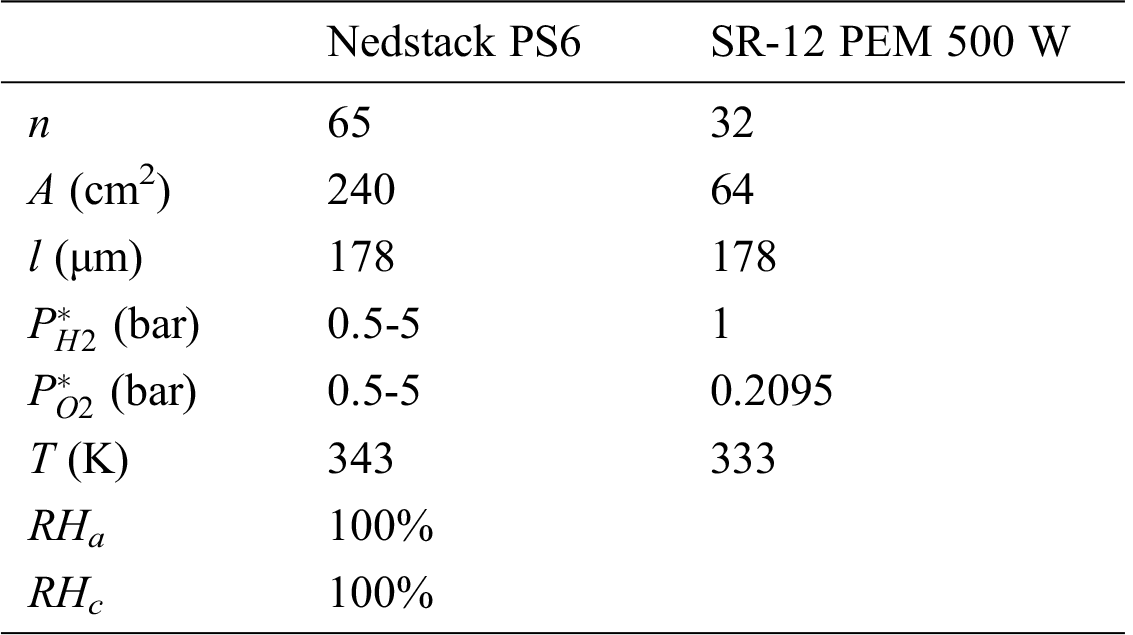To be fair in the comparison, the population size and maximum number of iterations for MFOA, PSO, and SCA are set to 30 (population size) and 100 (maximum number of iterations). During the optimization procedure, the RMSE between the calculated cell voltage and measured data is used in the fitness function, which needs to be the minimum. The unknown parameters of the PEMFC model are designated as decision variables. The lower and upper limits of these parameters are given in Tab. 2 [14,18].

Table 2: Lower and upper limits of the PEMFC model parametersThe best-identified parameters of PEMFC model applying MFOA, PSO, and SCA are shown in Tab. 3. To demonstrate the consistency of the considered optimizers, each one is executed 30 times. The statistical assessment of the considered optimizers for both types of the PEMFC is demonstrated in Tab. 4.

Table 3: Optimum parameters of fuel cells using different optimization algorithms.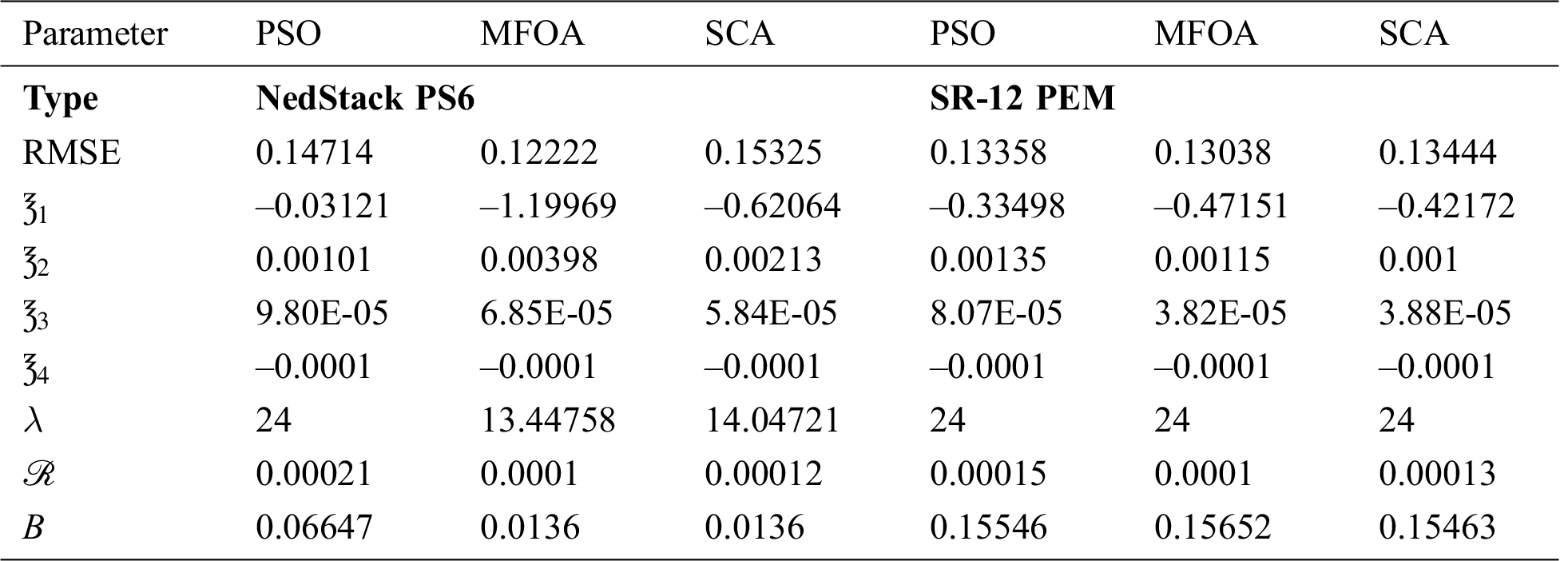Table 4: Statistical assessment of the considered optimizers (30 runs)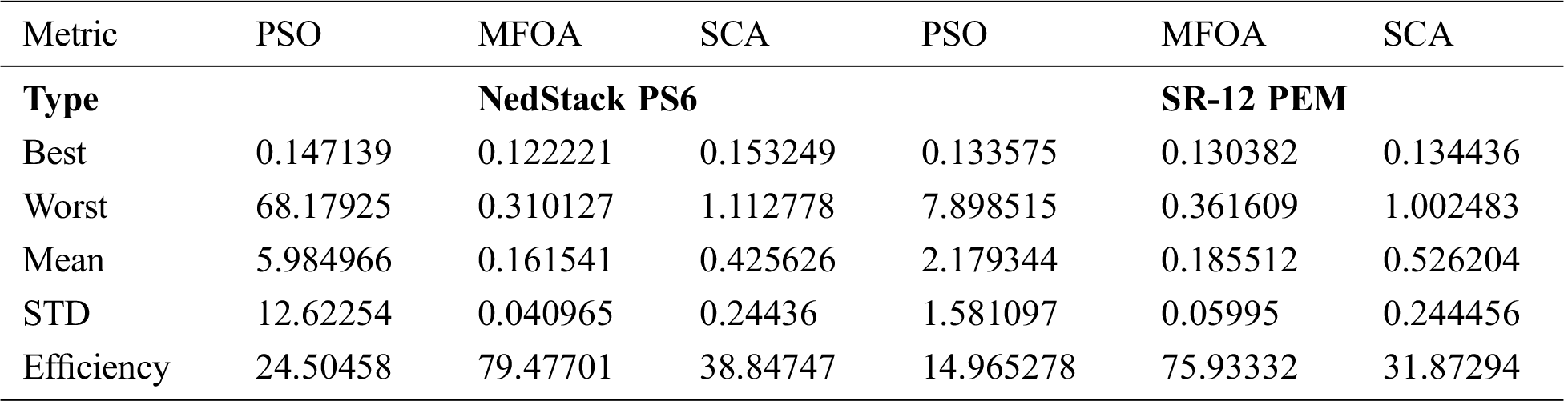Considering the data presented in Tab. 4, it can be observed that for both types of PEMFC, the MFOA has the top performance compared with PSO and SCA. For Nedstack PS6, the mean fitness function value ranged between 5.984966 and 0.161541. The smallest fitness of 0.161541 is reached using MFOA flowed by 0.425626 utilizing SCA. The lowest STD of 0.040965 is achieved by MFOA. The average efficiency values are 24.50458%, 79.477%, and 38.84747% for MFOA, PSO, and SCA, respectively. Regarding the second type of PEMFC (SR-12 PEM), the mean fitness function value ranged between 2.179344 and 0.185512. The smallest fitness of 0.185512 is reached using MFOA flowed by 0.526204 using SCA. The worst performance is allocated to the PSO method. The lowest STD of 0.05995 is achieved by MFOA. The average efficiency values are 14.965%, 79.933%, and 31.87%, respectively, for MFOA, PSO, and SCA. The details of 30 runs for two types of PEMFCs using considered optimizers are presented in Tab. 5. The variation of fitness functions through parameter identification of both fuel cells using different optimization are shown in Figs. 2 and 3.

Table 5: Details of 30 run for two types of PEMFCs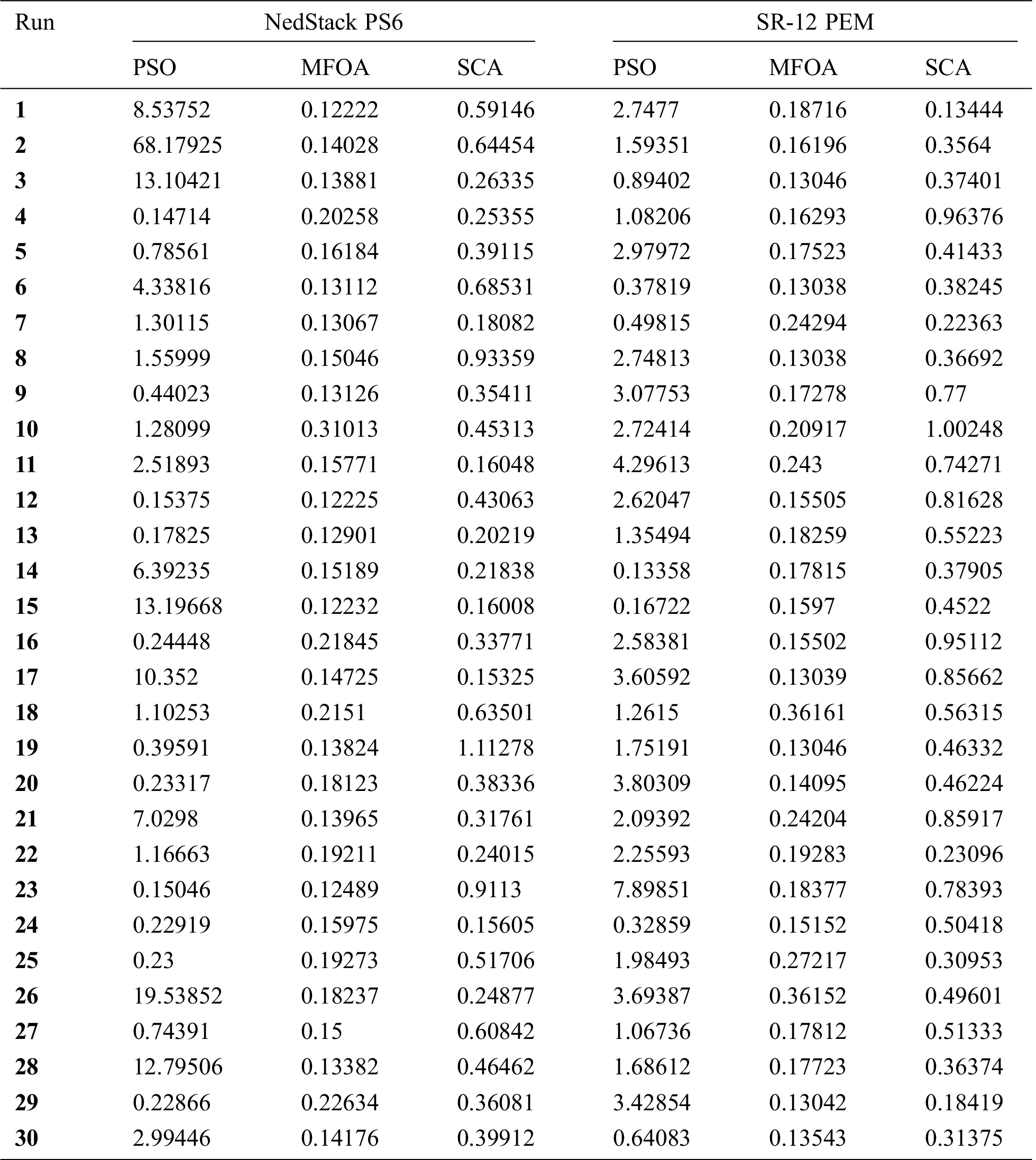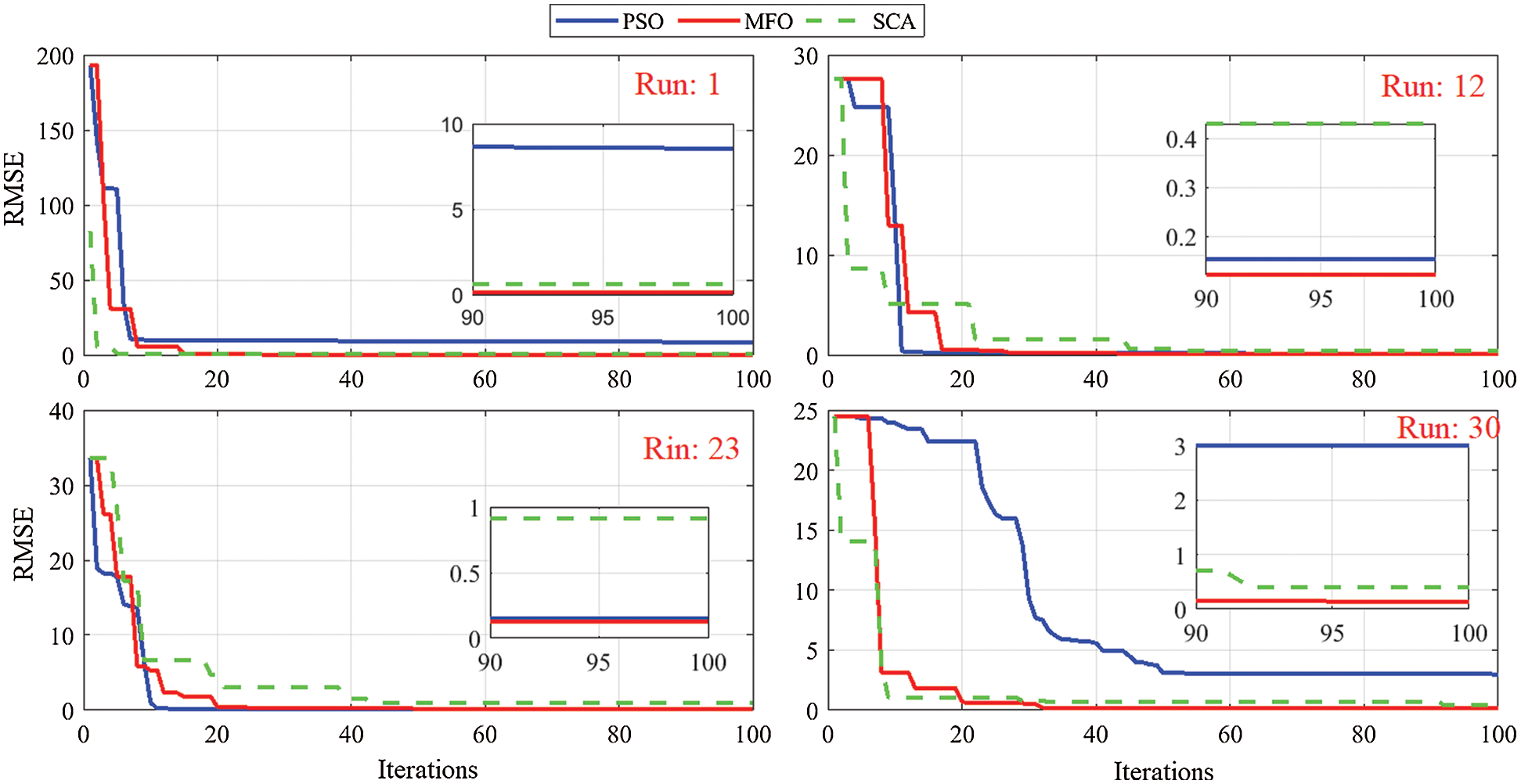Figure 2: Variation of fitness function through parameter identification of Nedstack PS6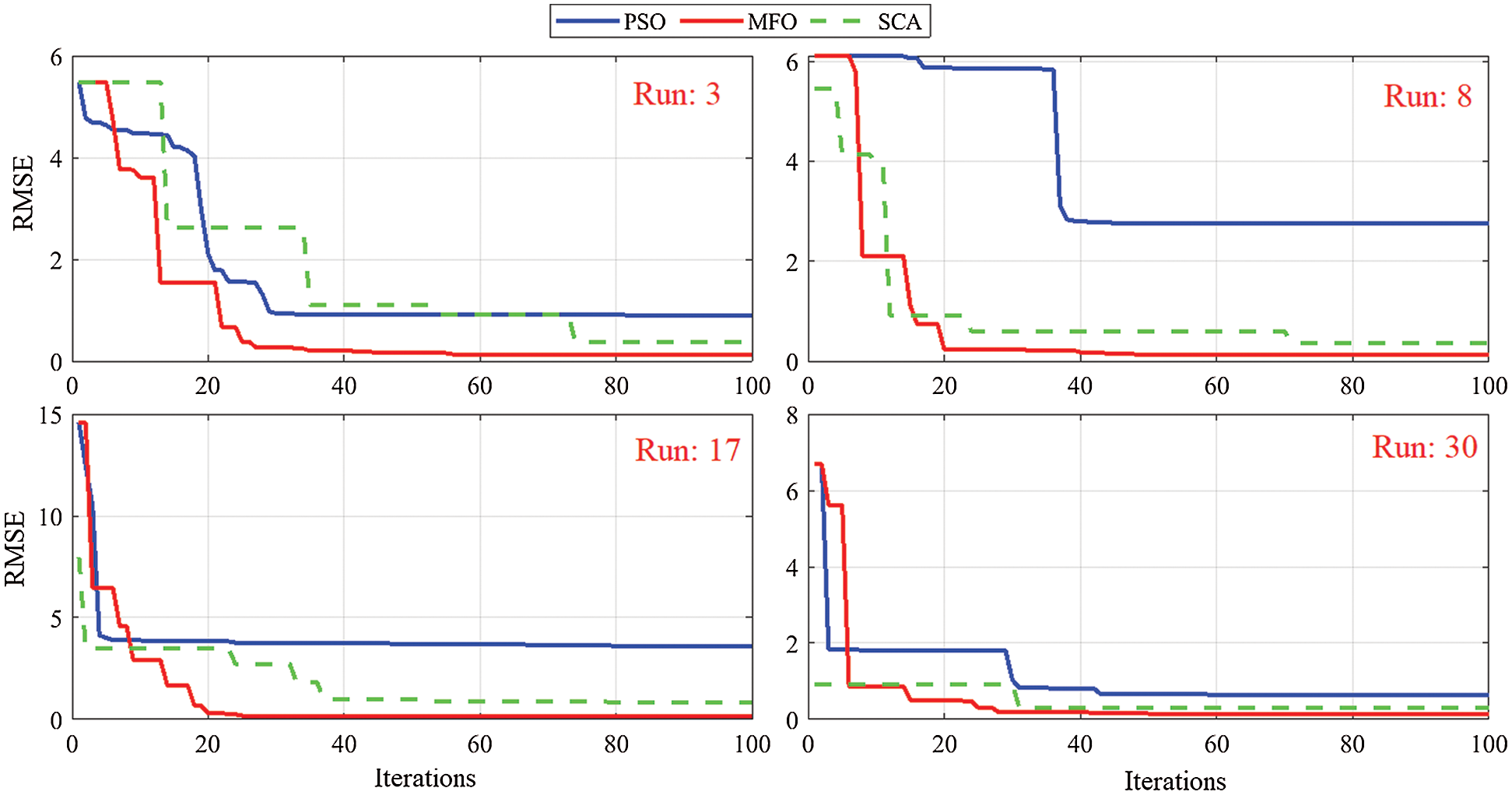Figure 3: Variation of fitness function through parameter identification of SR-12 PEM

A comparison between the estimated and measured data of NedStack PS6 is introduced in Fig. 4 (upper). There is high matching between the estimated dataset applying MFOA and the experimental data. The coefficient of determination and the mean absolute error values are 0.9984 and 0.2304, respectively. The variation of the absolute error is demonstrated in Fig. 4 (left down). The maximum absolute error is 0.57. This confirms the precision of the MFOA in determining the actual parameters of NedStack PS6. The result of the whiteness test for NedStack PS6 applying MFOA is displayed in Fig. 4 (right down). The key objective of such a test is to guarantee that the selected model parameters describe the measured data completely without any mismatch. It was determined using the residual autocorrelation function (RACF) at different time lags. Referring to Fig. 4 (right down), the RCAF values vary from −1 to +1.Figure 4: Fuel cell voltage, current, power, MAE and RCAF during parameter estimation of NedStack PS6 using MFOA

A comparison between the calculated and experimental dataset of SR-12 PEM is introduced in Fig. 5 (upper). There is high matching between the estimated dataset applying MFOA and the experimental data. The coefficient of determination and the mean absolute error values are 0.9979 and 0.2010, respectively. The absolute error is demonstrated in Fig. 5 (down left). The maximum absolute error is 0.56. This validates the precision of the MFOA in determining the actual parameters of SR-12 PEM. Referring to Fig. 5 (down right), the RCAF values vary from −1 to +1. Tab. 6 presents the details of measured, estimated and error of output voltage for both types of PEMFCs.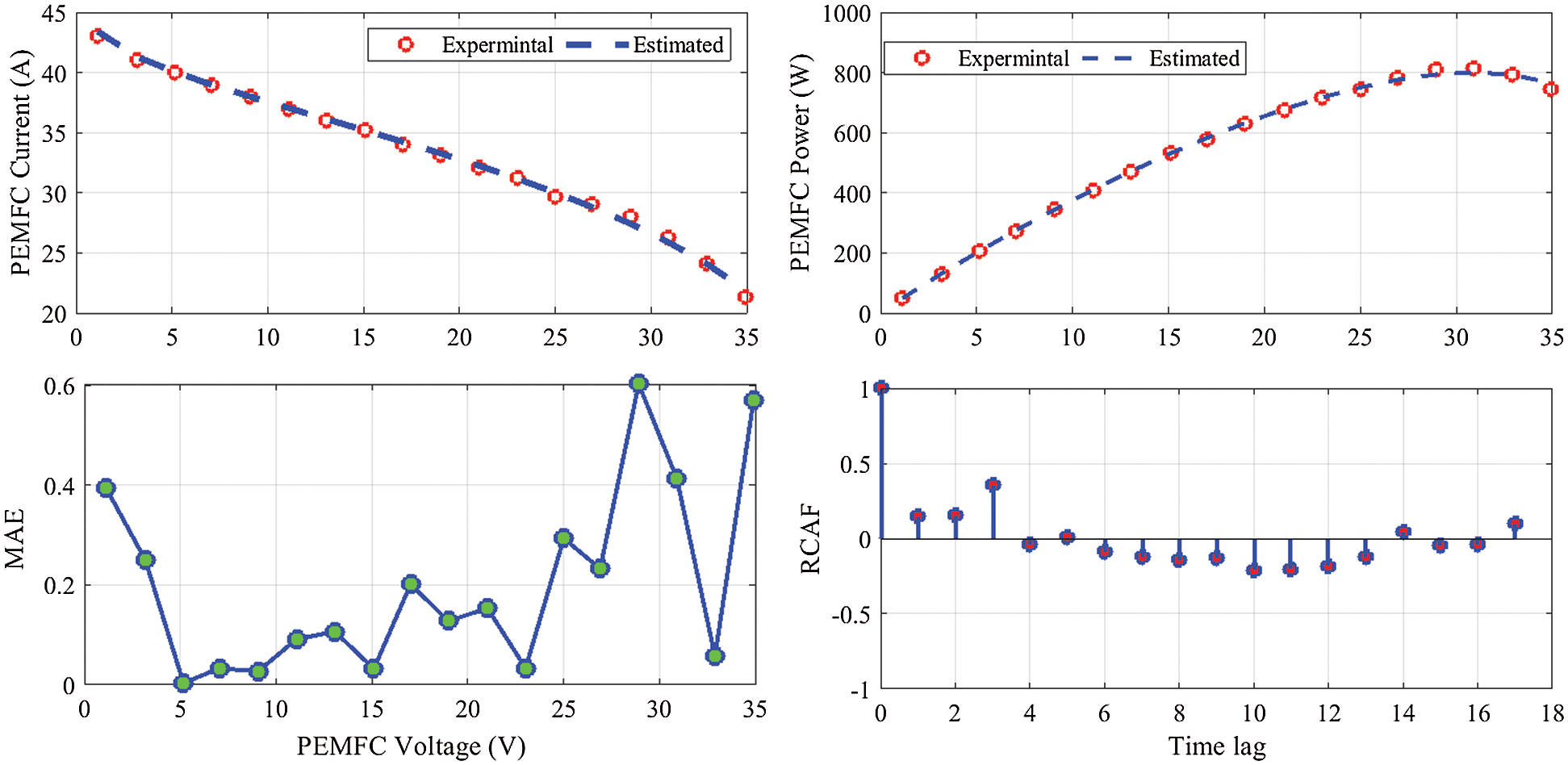Figure 5: Fuel cell voltage, current, power, MAE and RCAF during parameter estimation of SR-12 PEM applying MFOA

Table 6: Measured, estimated and error of PEMFC output voltage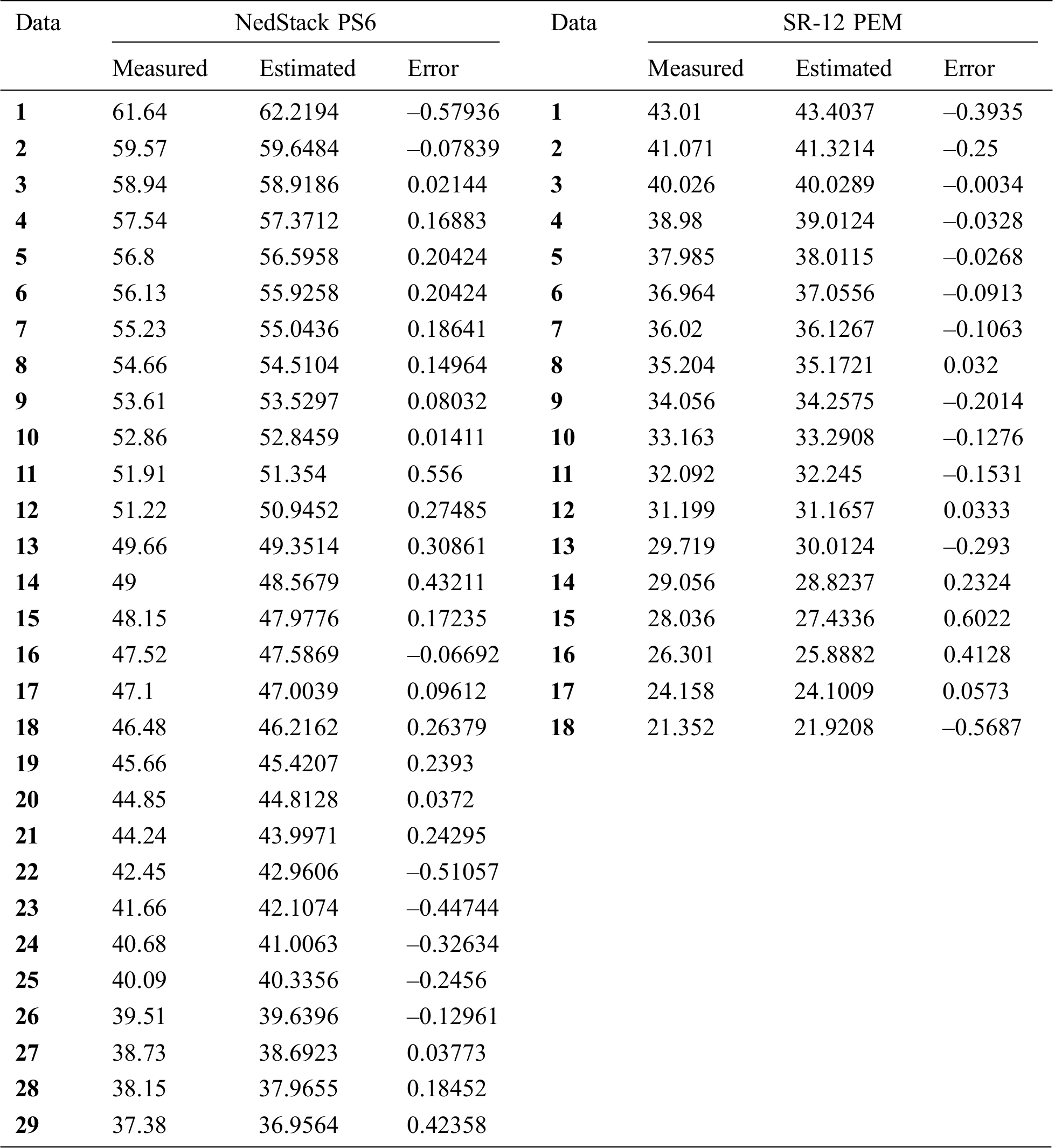5  Conclusion

In this research, a moth flame optimization algorithm (MFOA) has been used to estimate the seven unknown parameters (1, 2, 3, 4, λ, ℛ, and B) of the PEMFC model. Two types of PEMFCs, NedStack PS6 6 kW and SR-12 PEM 500 W are used to demonstrate the accuracy of the MFOA. The achieved polarization characteristics of the PEMFC model using MFOA were closely matched with the measured data. Four statistical metrics: standard deviation, RMSE, efficiency, and the coefficient of determination are used to compare the results obtained by MFOA with the sine cosine algorithm (SCA) and particle swarm optimization (PSO). For NedStack PS6, the mean fitness function values are 5.984966, 0.161541, and 0.425626, respectively, for MFOA, PSO, and SCA. The lowest STD of 0.040965 is achieved by MFOA. The efficiencies are 24.50458%, 79.477%, and 38.84747% for MFOA, PSO, and SCA, respectively. For PEMFC (SR-12 PEM), the smallest fitness of 0.185512 is reached using MFOA flowed by 0.526204 using SCA. The lowest STD of 0.05995 is achieved by MFOA. The average efficiency values are 14.965%, 79.933%, and 31.87% for MFOA, PSO, and SCA, respectively. These results confirm the superiority of MFOA compared with PSO and SCA in determining the unknown parameters of the PEMFC model. In the future, other types of PEMFCs will be considered to test the robustness of the MFOA.

Funding Statement: The authors received no specific funding for this study.

Conflicts of Interest: The authors declare that they have no conflicts of interest to report regarding the present study.

References

1.  1.  I. S. Sorlei, N. Bizon, P. Thounthong, M. Varlam, E. Carcadea et al., “Fuel cell electric vehicles—A brief review of current topologies and energy management strategies,” Energies, vol. 14, no. 1, pp. 252, 2021.
2.  2.  A. A. Kamel, H. Rezk and M. A. Abdelkareem, “Enhancing the operation of fuel cell-photovoltaic-battery-supercapacitor renewable system through a hybrid energy management strategy,” International Journal of Hydrogen Energy, vol. 46, no. 8, pp. 6061–6075, 2021.
3.  3.  A. Fathy, M. A. Abdelkareem, A. G. Olabi and H. Rezk, “A novel strategy based on salp swarm algorithm for extracting the maximum power of proton exchange membrane fuel cell,” International Journal of Hydrogen Energy, vol. 46, no. 8, pp. 6087–6099, 2021.
4.  4.  M. Aly, E. M. Ahmed, H. Rezk and E. A. Mohamed, “Marine predators algorithm optimized reduced sensor fuzzy-logic based maximum power point tracking of fuel cell-battery standalone applications,” IEEE Access, vol. 9, pp. 27987–28000, 2021.
5.  5.  A. Fathy, H. Rezk and T. M. Alanazi, “Recent approach of forensic-based investigation algorithm for optimizing fractional order PID based MPPT with proton exchange membrane fuel cell,” IEEE Access, vol. 9, pp. 18974–18992, 2021.
6.  6.  W. H. Tanveer, H. Rezk, A. Nassef, M. A. Abdelkareem, B. Kolosz et al., “Improving fuel cell performance via optimal parameters identification through fuzzy logic based-modeling and optimization,” Energy, vol. 204, no. 1, pp. 117976, 2020.
7.  7.  A. Fathy and H. Rezk, “Multi-verse optimizer for identifying the optimal parameters of PEMFC model,” Energy, vol. 143, no. 20, pp. 634–644, 2018.
8.  8.  F. Qin, P. Liu, H. Niu, H. Song and N. Yousefi, “Parameter estimation of PEMFC based on improved fluid search optimization algorithm,” Energy Reports, vol. 6, no. 10, pp. 1224–1232, 2020.
9.  9.  Z. Yuan, W. Wang, H. Wang and N. Razmjooy, “A new technique for optimal estimation of the circuit-based PEMFCs using developed Sunflower Optimization Algorithm,” Energy Reports, vol. 6, no. 1, pp. 662–671, 2020.
10. 10. Y. Cao, Y. Li, G. Zhang, K. Jermsittiparsert and M. Nasseri, “An efficient terminal voltage control for PEMFC based on an improved version of whale optimization algorithm,” Energy Reports, vol. 6, no. 1, pp. 530–542, 2020.
11. 11. E. A. El-Hay, M. A. El-Hameed and A. A. El-Fergany, “Optimized parameters of SOFC for steady state and transient simulations using interior search algorithm,” Energy, vol. 166, no. 40, pp. 451–461, 2019.
12. 12. A. S. Menesy, H. M. Sultan, A. Korashy, F. A. Banakhr, M. G. Ashmawy et al., “Effective parameter extraction of different polymer electrolyte membrane fuel cell stack models using a modified artificial ecosystem optimization algorithm,” IEEE Access, vol. 8, pp. 31892–31909, 2020.
13. 13. H. Nehrir, C. Wang and S. R. Shaw, “Fuel cells: Promising devices for distributed generation,” IEEE Power and Energy Magazine, vol. 4, no. 1, pp. 47–53, 2006.
14. 14. Z. J. Mo, X. J. Zhu, L. Y. Wei and G. Y. Cao, “Parameter optimization for a PEMFC model with a hybrid genetic algorithm,” International Journal of Energy Research, vol. 30, no. 8, pp. 585–597, 2006.
15. 15. S. Mirjalili, “Moth-flame optimization algorithm: A novel nature-inspired heuristic paradigm,” Knowledge-Based Systems, vol. 89, pp. 228–249, 2015.
16. 16. D. Gao, X. Li and H. Chen, “Application of improved particle swarm optimization in vehicle crashworthiness,” Mathematical problems in Engineering, vol. 6, no. 1, pp. 10–20, 2019.
17. 17. S. Mirjalili, “SCA: A sine cosine algorithm for solving optimization problems,” Knowledge-Based Systems, vol. 96, no. 63, pp. 120–133, 2016.
18. 18. I. Mohamed and N. Jenkins, “Proton exchange membrane (PEM) fuel cell stack configuration using genetic algorithms,” Journal of Power Sources, vol. 131, no. 1-2, pp. 142–146, 2004.This work is licensed under a Creative Commons Attribution 4.0 International License, which permits unrestricted use, distribution, and reproduction in any medium, provided the original work is properly cited.